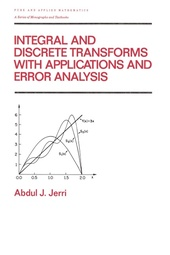# Integral and Discrete Transforms with Applications and Error Analysis

CRC Press
• 1. Auflage
• |
• erschienen am 18. November 2021
• |
• 848 Seiten

 E-Book | PDF ohne DRM | Systemvoraussetzungen
978-1-000-10431-8 (ISBN)

This reference/text desribes the basic elements of the integral, finite, and discrete transforms - emphasizing their use for solving boundary and initial value problems as well as facilitating the representations of signals and systems.;Proceeding to the final solution in the same setting of Fourier analysis without interruption, Integral and Discrete Transforms with Applications and Error Analysis: presents the background of the FFT and explains how to choose the appropriate transform for solving a boundary value problem; discusses modelling of the basic partial differential equations, as well as the solutions in terms of the main special functions; considers the Laplace, Fourier, and Hankel transforms and their variations, offering a more logical continuation of the operational method; covers integral, discrete, and finite transforms and trigonometric Fourier and general orthogonal series expansion, providing an application to signal analysis and boundary-value problems; and examines the practical approximation of computing the resulting Fourier series or integral representation of the final solution and treats the errors incurred.;Containing many detailed examples and numerous end-of-chapter exercises of varying difficulty for each section with answers, Integral and Discrete Transforms with Applications and Error Analysis is a thorough reference for analysts; industrial and applied mathematicians; electrical, electronics, and other engineers; and physicists and an informative text for upper-level undergraduate and graduate students in these disciplines.
 Auflage: 1. Auflage Sprache: Englisch Verlagsort: London | Großbritannien Verlagsgruppe: Taylor & Francis Ltd Zielgruppe: Für Beruf und Forschung | Für höhere Schule und Studium Schlagworte: ISBN-13: 978-1-000-10431-8 (9781000104318)weitere Ausgaben werden ermittelt
Abdul Jerri
Part 1 Compatible transforms: the method of separation of variables and the integral transforms; compatible transforms; classification of the transforms; comments on the inverse transforms - tables of the transforms; the compatible transform and the adjoint problem; constructing the compatible transforms for self-adjoint problems - second-order differential equations; the nth-order differential operator. Part 2 Integral transforms: Laplace transforms; Fourier exponential transforms; boundary and initial value problems - solutions by Fourier transforms; signals and linear systems - representation in the Fourier (spectrum) space; Fourier sine and cosine transforms; higher-dimensional Fourier transforms; the Hankel (bessel) tranforms; Laplace transform inversion; other important integral transforms. Part 3 Finite transforms - Fourier series and coefficients: Fourier (trigonometric) series and general orthogonal expansion; Fourier sine and cosine transforms; Fourier (exponential) transforms; Hankel (bessel) transforms; classical orthogonal polynomial transforms; the generalized sampling expansion; a remark on the transform methods and nonlinear problems. Part 4 Discrete transforms; discrete Fourier transforms; discrete orthogonal polynomial transforms; bessel-type poisson summation formula (for the Bessel-Fourier series and Hankel transforms). Appendix A: basic second-order differential equations and their (series) solutions - special functions. Appendix B: mathematical modeling of partial differential equations - boundary and initial value problems. Appendix C: tables of transforms.
Schweitzer Klassifikation
• Mathematik
•Allgemein
• Mathematik
•Analysis
•Funktionalanalysis
Thema Klassifikation
DNB DDC Sachgruppen
Dewey Decimal Classfication (DDC)
• Science
•Mathematics
•Analysis
BIC Classifikation
BISAC ClassifikationDateiformat: PDF
Kopierschutz: ohne DRM (Digital Rights Management)

Systemvoraussetzungen:

Computer (Windows; MacOS X; Linux): Verwenden Sie zum Lesen die kostenlose Software Adobe Reader, Adobe Digital Editions oder einen anderen PDF-Viewer Ihrer Wahl (siehe E-Book Hilfe).

Tablet/Smartphone (Android; iOS): Installieren Sie die kostenlose App Adobe Digital Editions oder eine andere Lese-App für E-Books (siehe E-Book Hilfe).

E-Book-Reader: Bookeen, Kobo, Pocketbook, Sony, Tolino u.v.a.m. (nur bedingt: Kindle)

Das Dateiformat PDF zeigt auf jeder Hardware eine Buchseite stets identisch an. Daher ist eine PDF auch für ein komplexes Layout geeignet, wie es bei Lehr- und Fachbüchern verwendet wird (Bilder, Tabellen, Spalten, Fußnoten). Bei kleinen Displays von E-Readern oder Smartphones sind PDF leider eher nervig, weil zu viel Scrollen notwendig ist. Ein Kopierschutz bzw. Digital Rights Management wird bei diesem E-Book nicht eingesetzt.

Weitere Informationen finden Sie in unserer E-Book Hilfe.